Courses
Courses for Kids
Free study material
Free LIVE classes
More

# Halves and Quarters Class 4 Notes CBSE Maths Chapter 9 (Free PDF Download)LIVE
Join Vedantu’s FREE Mastercalss

## Halves and Quarters Revision Notes and Worksheets for Quick and Easy Preparation

Class 4 is a crucial stage for the development of mathematical concepts related to fractions. Chapter 9 deals with the concepts of Halves and Quarters, and the basics of fractions. Students are taught how an object can be halved or quartered using certain methods.

To understand these concepts, download and refer to Halves and Quarters revision notes prepared by the top subject specialist of Vedantu. The simplest explanation of these basic fractions will help students to build a concept for complex fractions later.

Last updated date: 20th Sep 2023
Total views: 143.7k
Views today: 3.43k

## Access Class - 4 Mathematics Chapter - 9 Halves and Quarters Revision Notes

### Half-Half:

• Half-Half means dividing a whole thing into two equal parts each part is known as half.

• Examples: We can divide an apple into two equal parts where each part is known as half and both halves can be counted.

• Halves of Various shapes: In the below image all three things are divided into two equal halves. Note that two equal halves make one whole object.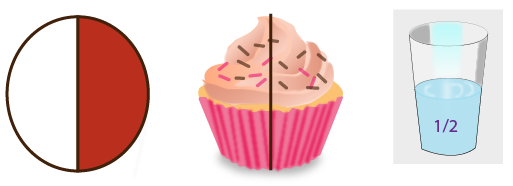Image: Half shapes

• Representation of half in fraction form:  Half is written as $\frac{1}{2}$ in fraction form. It generally means  1 part out of 2.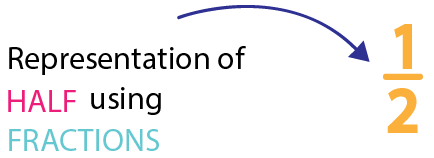Image: Representation of half in fraction form

### Properties of Half

• Adding two halves makes one.

• By dividing the number by 2 we can get half of any number.

• In decimal numbers, half is denoted by 0.5.

• We can find half of a number by multiplying it by $\frac{1}{2}$.  For example, if we 6 chips packets then half of it can be obtained by $6\times \frac{1}{2}=3$

### Solved Examples

1: There are 88 students in a classroom, and half of them are boys. Find the number of girls in the classroom?

Ans: Total number of students in a class = 88

It is mentioned that half of the students are boys. So that means two get the half value. We have to divide the whole number by 2 to get the other half value as given

$\Rightarrow \frac{88}{2}=44$

Therefore the result is 44. It means that there are 44 boys and 44 girls present in the classroom.

Many Ways to Cut into Half:

• We can cut an object into two equal halves in different ways.

• Example: In the given image below we can see that a square has been divided into two equal parts in different ways.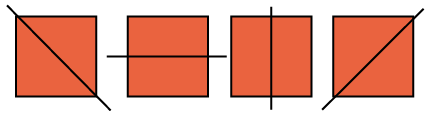Image: Many ways to cut into Half

Half of Many Pieces:

• After dividing one thing/object into two equal parts. Again each half can be divided into many pieces.

2: Krithi has two parts of diary milk chocolate. But she wants to share the chocolate with her 8 friends equally. What can Krithi do?

Ans: If we observe the given chocolate it has been divided into 2 parts where each part contains 4 pieces. So now Krithi can divide the 2 parts of the chocolate into 8 equal parts by which she can share all 8 equal pieces with her friends.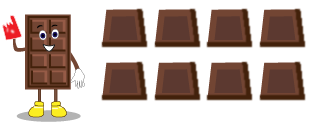Image: Chocolate

Quarter:

• Dividing one object into four equal parts where each part is known as Quater.

• We can also get the quarter by dividing the two halves of one whole object into two more equal parts. Here each part is known as one-quarter of the given object.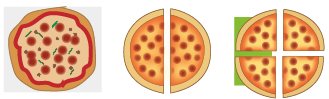Image: Quarter

• Given below images shows how images are divided to get the quarter part.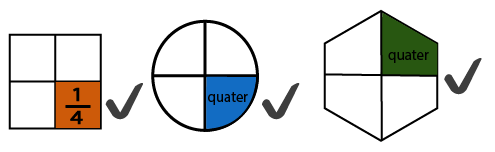Image: Quarter part

• f quarter in fraction form:  A quarter is written as $\frac{1}{4}$in fraction form. It generally means  1 part out of 4.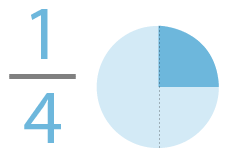Image: ¼ part of circle

3: Shruthi is in the market and has only Rs 70 with her. Shruthi wants to buy $\frac{1}{4}$ kg mangoes and $\frac{1}{4}$ kg apples. Can she buy all the things on her shopping list? Kindly help her in calculating the total amount?

Ans: Total money Keerthi has = Rs 70

Her shopping list includes = $\frac{1}{4}$ kg mango and $\frac{1}{4}$ kg apples.

Cost of 1 kg mango = Rs 100

Cost of $\frac{1}{4}$ kg of grapes = Rs. $\frac{1}{4}-25$

Cost of 1 kg apple = Rs 80

Cost of $\frac{1}{4}$ kg apple = Rs  $\frac{1}{4}80-20$

Total cost of all the fruits = 25 + 20

Therefore total cost of fruits = Rs 45

Hence, Keerthi can buy all the fruits in her shopping list.

Many Ways to Make a Quarter:

• We can cut the object in many ways to make a quarter. The below image gives an idea of different ways of cutting to get equal quarters.

• Example: When you have a sandwich to share with your friends equally try out the different ways mentioned below.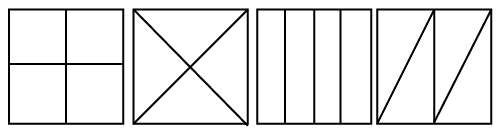Image: Many ways to Make a quarter

Half and Quarter in Metres:

• Metre is the basic unit of length (or distance) in the Metric System. It is used to measure the length. Here 1 metre is represented as 1m.

 Remember:1m=100cm
• Half of a metre is nothing but dividing the metre into two equal parts. So Half of the metre is represented by $\frac{1}{2}m$.

• A quarter of a metre is nothing but dividing the metre into four equal parts. So a quarter of a metre is represented by $\frac{1}{4}m$.

• We know that 1m = 100cm

Now,

$\frac{1}{2}m=50$cm

$\frac{1}{4}m=25$cm

### Half and Quarter in Litres:

• A litre  is one of the metric units of volume. Mostly we use litres to measure the liquid form. Here 1 metre is represented as 1l.

 Remember: 1 litre =1000 millilitres
• Half of a litre  is nothing but dividing the litre into two equal parts. So the half of the litre is represented by $\frac{1}{2}l$.

• A quarter of a litre is nothing but dividing the litre into four equal parts. So a quarter of a litre is represented by $\frac{1}{4}l$.

• We know that 1 litre = 1000 millilitres

Now,

$\frac{1}{2}\text{liter}=500\;\text{millilitres}$

$\frac{1}{4}\text{liter}=250\;\text{millilitres}$

### Practise Questions

1. If the cost of half of the jackfruits is 50 rupees then what will be the cost of full jackfruit?
Ans: 100 rupees

2. Oliva has 100 chocolates. But she wants to know what is the quarter of them?

Ans: 25 Chocolates

## Importance of CBSE Class 4 Maths Chapter 9 Halves and Quarters

This chapter has an innovative way of describing what fractions are to the students. By depicting a story where a monkey is unable to divide a chapati into two halves, this chapter introduces the concept of fractions. Check these points to understand the importance of Class 4 Maths Chapter 9 for students.

• Students will realise what a half and a quarter stand for. They will also understand what half of half means and how it is similar to a quarter.

• The concept of Halves and Quarters Class 4 will enable kids to develop a conceptual foundation for learning fractions in future classes. At this stage, this primitive concept is good for explaining two basic fractions.

• They will learn how to solve division sums for Class 4 with answers. In fact, they will also learn that only symmetric objects can be divided into equal halves and quarters.

• Students will also realise how an object is not the half of a bigger object as both halves have to be equal to each other.

• Using the learnt concepts of Halves and Quarters Class 4, students will be able to solve arithmetic problems such as grocery lists, vegetable buying, etc.

## Benefits of Vedantu’s Halves and Quarters Worksheets and Revision Notes

• Experts have explained the concepts in the revision notes in a simpler way. By referring to these notes for the fraction Chapter for Class 4, you will be able to understand what halves and quarters stand for and how to determine them.

• Make your concepts related to symmetric objects and shapes clearer using the explanations in these notes.

• Find out ‘how to cut a shape into half?’ is given in the exercise by following the Halves and Quarters Class 4 solutions.

• Recall and brush up on your division skills by solving the division sums for Class 4 present in this chapter exercises and worksheets.

## Make Your Fraction Foundation Strong with Chapter 9 Revision Notes

Download the fraction worksheet for Class 4 and revision notes from Vedantu and complete your study material for this chapter. Learn how the experts have explained the concepts and framed the answers to all questions in the worksheets. Refer to the NCERT Solutions for Class 4 Maths Chapter 9 for this chapter and prepare well for the upcoming exams.

## FAQs on Halves and Quarters Class 4 Notes CBSE Maths Chapter 9 (Free PDF Download)

1. Is a half bigger than a quarter?

A half is represented by ½ and a quarter is represented by ¼. We have studied that if the denominator is bigger in one fraction than the other one, then the former one is smaller. Here denominator 4 is bigger than denominator 2. Hence, a half is bigger than a quarter.

2. How can I experiment to find out whether a half is bigger than a quarter?

If you tear a page into two halves and then tear half of the page into two halves, you will get a quarter of that full page.

3. How can I study this chapter?

Study the concepts and solve the exercise questions first. Practice using the worksheets and revise the notes before an exam.